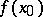# Non-standard analysis

(diff) ← Older revision | Latest revision (diff) | Newer revision → (diff)
A branch of mathematical logic concerned with the application of the theory of non-standard models to investigations in traditional domains of mathematics: mathematical analysis, function theory, the theory of differential equations, probability theory, and others. The basic method of non-standard analysis can roughly be described as follows. One considers a certain mathematical structureand constructs a first-order logico-mathematical language that reflects those aspects of this structure that are of interest to the investigator. Then one constructs by methods of model theory a non-standard model of the theory ofthat is a proper extension of. Under a suitable construction new, non-standard, elements of the model can be interpreted as limiting "ideal" elements of the original structure. For example, if as the original structure one takes the field of real numbers, then it is natural to treat the non-standard elements of the model as "infinitesimals" , that is, as infinitely large or infinitely small, but non-zero, real numbers. Then all the usual relations between real numbers carry over to the non-standard elements, with the preservation of all their properties that can be expressed in the logico-mathematical language. Similarly, in the theory of filters on a given set the intersection of all non-empty elements of the filter determines a non-standard element; in topology this gives rise to a family of non-standard points situated "infinitely close" to a given point. The interpretation of the non-standard elements of a model often makes it possible to give convenient criteria for ordinary concepts in terms of non-standard elements. For example, it can be proved that a standard real-valued functionis continuous at a standard pointif and only ifis infinitely close tofor all (non-standard) pointsinfinitely close to. The criterion thus obtained can be successfully applied to proofs of ordinary mathematical results.